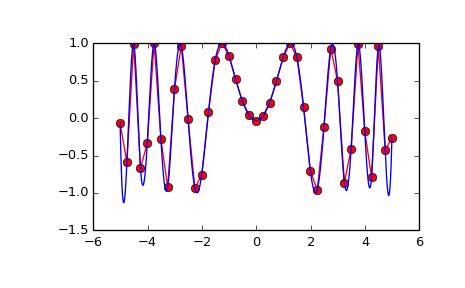# scipy.interpolate.interp2d¶

class scipy.interpolate.interp2d(x, y, z, kind='linear', copy=True, bounds_error=False, fill_value=nan)[source]

Interpolate over a 2-D grid.

x, y and z are arrays of values used to approximate some function f: z = f(x, y). This class returns a function whose call method uses spline interpolation to find the value of new points.

If x and y represent a regular grid, consider using RectBivariateSpline.

Parameters: x, y : array_like Arrays defining the data point coordinates. If the points lie on a regular grid, x can specify the column coordinates and y the row coordinates, for example: >>> x = [0,1,2]; y = [0,3]; z = [[1,2,3], [4,5,6]]  Otherwise, x and y must specify the full coordinates for each point, for example: >>> x = [0,1,2,0,1,2]; y = [0,0,0,3,3,3]; z = [1,2,3,4,5,6]  If x and y are multi-dimensional, they are flattened before use. z : array_like The values of the function to interpolate at the data points. If z is a multi-dimensional array, it is flattened before use. The length of a flattened z array is either len(x)*len(y) if x and y specify the column and row coordinates or len(z) == len(x) == len(y) if x and y specify coordinates for each point. kind : {‘linear’, ‘cubic’, ‘quintic’}, optional The kind of spline interpolation to use. Default is ‘linear’. copy : bool, optional If True, the class makes internal copies of x, y and z. If False, references may be used. The default is to copy. bounds_error : bool, optional If True, when interpolated values are requested outside of the domain of the input data (x,y), a ValueError is raised. If False, then fill_value is used. fill_value : number, optional If provided, the value to use for points outside of the interpolation domain. If omitted (None), values outside the domain are extrapolated. values_x : ndarray, shape xi.shape[:-1] + values.shape[ndim:] Interpolated values at input coordinates.

See also

RectBivariateSpline
Much faster 2D interpolation if your input data is on a grid
BivariateSpline
a more recent wrapper of the FITPACK routines
interp1d
one dimension version of this function

Notes

The minimum number of data points required along the interpolation axis is (k+1)**2, with k=1 for linear, k=3 for cubic and k=5 for quintic interpolation.

The interpolator is constructed by bisplrep, with a smoothing factor of 0. If more control over smoothing is needed, bisplrep should be used directly.

Examples

Construct a 2-D grid and interpolate on it:

>>> from scipy import interpolate
>>> x = np.arange(-5.01, 5.01, 0.25)
>>> y = np.arange(-5.01, 5.01, 0.25)
>>> xx, yy = np.meshgrid(x, y)
>>> z = np.sin(xx**2+yy**2)
>>> f = interpolate.interp2d(x, y, z, kind='cubic')


Now use the obtained interpolation function and plot the result:

>>> import matplotlib.pyplot as plt
>>> xnew = np.arange(-5.01, 5.01, 1e-2)
>>> ynew = np.arange(-5.01, 5.01, 1e-2)
>>> znew = f(xnew, ynew)
>>> plt.plot(x, z[0, :], 'ro-', xnew, znew[0, :], 'b-')
>>> plt.show()Methods

 __call__(x, y[, dx, dy, assume_sorted]) Interpolate the function.

#### Previous topic

scipy.interpolate.Rbf.__call__

#### Next topic

scipy.interpolate.interp2d.__call__